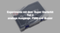This article is about how to output analog values, for example to vary the brightness of an LED or to change the color of an RGB LED. It will also be shown how to generate sounds with the microcontroller.

### Required Hardware

 Number Component Note 1 Super Starter Kit 1 9V block battery or 9V power supply unit optional

### Analog outputs

Actually the ATMEGA328P has only digital outputs. That means the voltage at the output is either 0 V or 5 V. However, it is also possible to generate analog signals with digital outputs by switching them on and off again and again. The ratio of the on-time to the off-time then determines the analog value. If the output is continuously switched on, the output voltage is 5 V. If it is continuously switched off, the output voltage is 0 V. If the output is switched on and off for the same time, the average value of the output voltage is 2.5 V. Since the pulse width determines the analog value, this method is called pulse width modulation (PWM). The ATMEGA328P can be controlled with the function analogWrite(pin,value); to write analog values to a pin. The pin must be written as in digitalWrite(pin,value); with the function pinMode(pin, OUTPUT); can be defined as output. The specified value (value) can be between 0 and 255. The duty cycle is thereby changed between 0 and 100%.

With the ATMEGA328P only six of the pins can be used for PWM. These are pins 5 and 6 with a frequency of about 1 kHz and pins 9, 10, 11 and 3 with a frequency of about 500 Hz. The timers of the microcontroller are used to generate the PWM signal. For pins 5 and 6 this is timer0, for pins 9 and 10 it is timer1 and for pins 11 and 3 it is timer2. If the timers are also used elsewhere it can lead to conflicts.

Timer0 is used for the internal millisecond counter, therefore the PWM frequency for pins 5 and 6 is equal to 1 kHz. As long as you don't want to change the PWM frequency, the millisecond counter and PWM on pins 5 and 6 can be used simultaneously without any problems.

The timer2 is used for sound generation. A description of this function will be given later in this article. For tone generation, the frequency of the timer is changed and the duty cycle should be 50%. Here a simultaneous use with PWM is not possible.

To get an approximate DC voltage, the energy delivered during the on-time must be stored and delivered again during the off-time. This is done most simply with a resistor and a capacitor. Such a circuit is called a RC element.While the output is at 5 V, the capacitor is charged via the resistor and while it is at 0, it is discharged again via the resistor.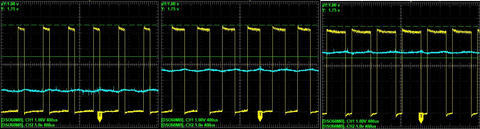The picture shows the PWM signal at the input (yellow line) and the average value at the output of the RC element (blue line) for 25%, 50% and 75%.

The product R * C is called the time constant of the RC element. This is the time needed to charge the capacitor to 63.2% of the maximum voltage. For a good screening effect, the time constant should be at least ten times as large as the period of the PWM signal. So for 1 kHz 10 ms are required. With a resistor of 10 kΩ this results in a capacitance of
10-2 s / 104 Ω = 10-6 F or 1 µF. The following figure shows the sieving effect with a capacitor of 0.1 µF = 1 ms and a capacitor of 1 µF = 10 ms.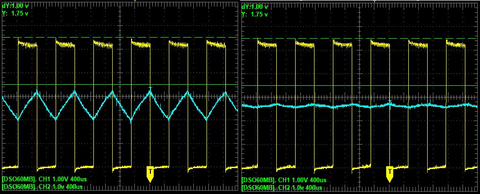With a higher time constant, the sieving effect can be further improved. However, this results in a delayed response to changes in the average value. The following figure shows the output voltage when every 0.1 s alternating 0 and 128 with analogWrite(pin,value) is output. You can clearly see the exponential increase or decrease.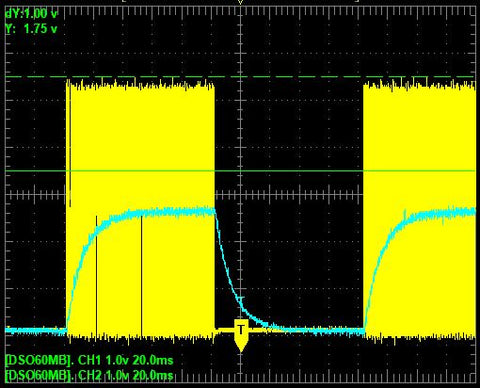Another possibility to form the average value is an inductance. In this case not the voltage but the current is stored. When switched on, the current increases exponentially and decreases the same when switched off. Just as an average voltage was reached at the capacitor, an average current is reached at the inductor. This is used in DC motors to control the speed with the PWM signal.

A third possibility is to use the inertia of the eye to control the brightness. If you control a light emitting diode with the PWM signal, it is able to react immediately. This means that the light emitting diode generates light flashes with full brightness, whereby the duration of the light flash depends on the pulse duration of the PWM signal. However, the human eye cannot follow this fast change, but perceives a more or less bright glow depending on the pulse duration.

Dimming an LED with PWM

The circuit is quite simple. As with the traffic light in the first part, the LED is connected to the output pin of the microcontroller via a 1 kΩ resistor. This time pin 5 is chosen because it supports PWM. The PWM frequency is 970 Hz.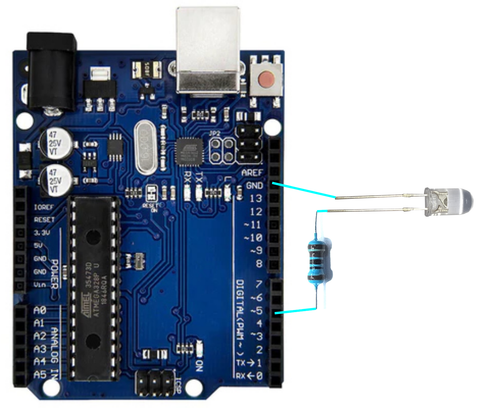The LED should slowly get brighter and brighter and after reaching a certain maximum value it should get dimmer again. Since between 50% and 100% the brightness change is hardly noticeable, 128 is used as maximum value. The sketch for this is very simple.

#define LEDPIN 5
#define MAX 128

First two constants are defined. One for the used pin number and the other for the maximum output value

int value = 0;
boolean on = true;

In the global integer variable value the current output value is stored. The initial value is 0. The boolean variable at holds whether the value should be incremented or not.

void setup() {
pinMode(LEDPIN, OUTPUT);
}

In the setup function only pin 5 is defined as output

void loop() {
analogWrite(LEDPIN, value);

In the main loop first the current value is written with analogWrite(pin, value); is output. This is followed by a condition which is set with the command if command. This is followed by the condition in parentheses. Conditions always have a boolean value, that is, they are true or false. The global variable at can therefore be used directly as a condition. All commands within the following looped brackets are only executed if the condition is true. In this case, if the value is to be increased. The command value++; is a shortened notation of the command value = value + 1; increases the value by 1. Now follows another condition. If the content of the variable value exceeds the maximum value, the direction is to be switched. to = false; and the variable value should be set to the maximum value.

if (to) {
value ++;
if (value > MAX) {
on = false;
value = MAX;
}
}

With the command else starts a part of the program that is only executed if the condition in the associated if command is not fulfilled. This applies again to all commands within the following looped brackets. In this case, if the value is to be decreased. The command value- -; is a shortened notation of the command value = value - 1; decreases the value by 1. Now follows another condition. If the content of the variable value is less than zero, the direction is to be switched. to = true; and the variable value must be set to 0.

else
{
value --;
if (value < 0) {
value = 0;
up = true;
}
}

Finally, a delay of 50ms is added and the function loop() is terminated with the looped parenthesis.

delay(50);
}

RGB - light emitting diode

The set also includes a so-called RGB light emitting diode.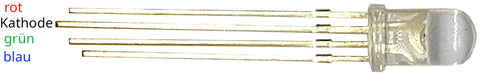In an RGB led, three light-emitting diodes are housed in one case. One red, one green and one blue. To keep the number of connecting wires small, either the anodes or the cathodes of all diodes are combined. The RGB-Led in the set has a common cathode.The longest connecting wire is the cathode, the middle two are red and green, and the shortest is blue. As with the simple light emitting diode, series resistors are required to limit the current.

Play of colors with RGB - Led

As an example sketch, an RGB led is to be controlled so that the color changes randomly. For this purpose, the RGB-Led is connected to pins 9, 10 and 11 of the microcontroller via three 1 kΩ resistors. These three pins support PWM, so they can be used as analog outputs. The circuit then looks like this.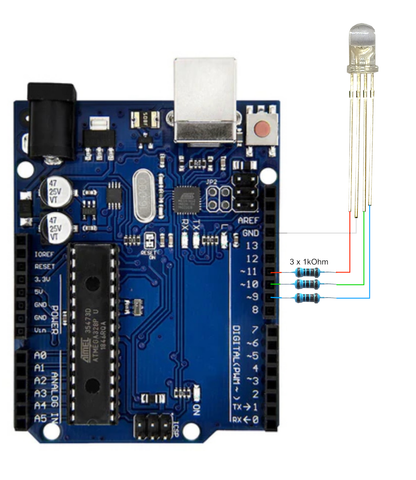In the sketch, three global variables are used to store the current values for red, green and blue. In the main loop these three values are output to the corresponding pins. After that one of the three variables is changed randomly.

At the beginning the used pins are defined as constants. Furthermore the constant DELTA is defined. This is used to change the value of a color. The larger this value, the more abrupt the color change.

#define PINROT 11
#define PINGRUEN 10
#define PINBLUE 9
#define DELTA 4

Three global variables for the current color values. Value range 0 to 255.

int red;
int green;
int blue;

In the setup() function, the three pins are defined as output and the color values are set to their initial value.

void setup() {
pinMode(PINROT, OUTPUT);
pinMode(PINGRUEN, OUTPUT);
pinMode(PINBLUE, OUTPUT);
red = 128;
green = 128;
blue = 128+9;
}

In the main loop the output and the change of the values is done. First the values are transferred to the pins

void loop() {
analogWrite(PINROT, red);
analogWrite(PINGRUEN, green);
analogWrite(PINBLUE, blue);

With the function random(MAX) a random number between 0 and MAX - 1 is generated. There is also a second variant random(MIN, MAX) which generates a random number between MIN and MAX - 1 are generated. With the first call the values 0 or 1 are obtained. This value is stored in the local variable at stored. This controls whether a value is to be increased or decreased. The second call returns the numbers 0, 1 or 2. The result is stored in the local variable color and is used to select the color value to be changed.

boolean on = random(2);
int color = random(3);

The following program line contains a conditional assignment:
VARIABLE = CONDITION?VALUE1:VALUE2;
The variable is assigned the value1 if the condition is met, otherwise the value2. In the following case the local variable d is assigned the value of the constant DELTA is assigned when to has the value 1, otherwise the negative value of the constant DELTA
int d = (on)?DELTA:-DELTA;

Now depending on the content of the variable color the corresponding color value should be changed. This could be achieved by several conditions. However, more elegant and clearer is the switch command. After the keyword switch is followed by the variable to be examined in parentheses. Only whole numbers or letters are allowed here. Then the individual cases follow in looped brackets. Each case begins with the keyword case followed by the value that the variable must have for the case to occur. The colon is followed by the commands to be executed when the case occurs. The command break; at the end causes an exit from the switch command. The last case can also be used in place of case x : with default : can be defined. This means that the code after the colon is always executed if none of the preceding cases has occurred. This code will be executed even if a preceding case has not occurred with break; has been terminated.
The command red += d; is a shortened notation for red = red + d;

switch(color) {
case 0: red += d; break;
case 1: green += d; break;
case 3: blue += d; break;
}

Since the changes can also cause values outside the allowed value range, the color value must be corrected to the corresponding limit value in such a case

if (red < 0) rot = 0;
if (rot >255) red = 255;
if (green < 0) gruen = 0;
if (gruen >255) green = 255;
if (blue < 0) blau = 0;
if (blau >255) blue = 255;

After a delay of 10 ms the function of the main loop is terminated with a looped bracket.

delay(10);
}

If you hold a piece of thin white paper in front of the diode, you can see the color change better.

### Generate tones

At the end of this part, I will describe the two piezo buzzers present in the set. These are small speakers that use the fact that a piezoelectric crystal bends when voltage is applied. This bending is transferred to a diaphragm, which then generates the sound waves. The frequency range is between 300 Hz and 20 kHz. The buzzers are loudest at around 2 to 3 kHz.One of the buzzers, the one with the sticker, is an active buzzer. It has an internal tone generator. As soon as voltage is applied, it produces a whistling tone at 2.5 kHz and a sound level of about 60 dB. To use it, the sticker must be removed. The polarity must be observed. The plus pole is on the side of the tab of the sticker. The positive pole is also marked on the top of the housing.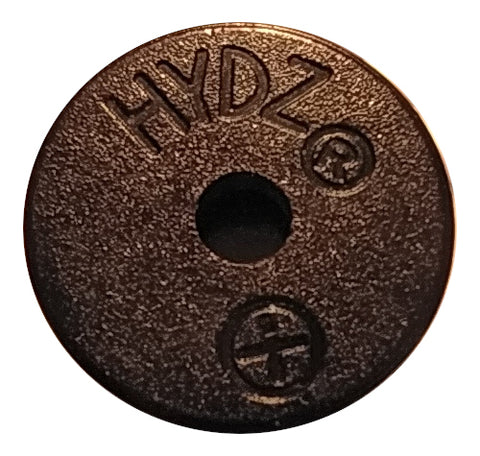The control is the same as for a LED with digitalWrite(PIN, VALUE); where at value 1 the buzzer whistles and at value 0 it is quiet. The example sketch generates an alarm signal with the active buzzer. The buzzer is connected to the positive terminal on pin 4 and to GND.

The program is simple and does not contain any new commands. In the setup function the pin is defined as output and set to 0. In the main loop the buzzer is switched on for 100 ms and then switched off for 60 ms. Then it is switched on for 600 ms and switched off for 100 ms. This sequence is repeated continuously.

#define BUZZERPIN 4
void setup() {
pinMode(BUZZERPIN, OUTPUT);
digitalWrite(BUZZERPIN, 0);
}
void loop() {
digitalWrite(BUZZERPIN, 1);
delay(100);
digitalWrite(BUZZERPIN, 0);
delay(60);
digitalWrite(BUZZERPIN, 1);
delay(600);
digitalWrite(BUZZERPIN, 0);
delay(100);
}

The other buzzer in the set is passive and can be used practically like a loudspeaker. The microcontroller has to generate the sound here. The frequency of the tone can be changed, unlike the active buzzer. To output a sound the Arduino IDE has the command tone(PIN, FREQUENCY); With this command a square wave signal with the specified frequency is output at the specified pin.

As an example a siren is to be realized, which constantly increases the frequency up to a maximum value and then decreases it again to a minimum value. Pin 4 is used again. The connection is the same as for the active buzzer.

First the pin used, the minimum frequency, the maximum frequency and the value by which the frequency is to be increased or decreased are defined as constants.

#define BUZZERPIN 4
#define FMIN 800
#define FMAX 5000
#define DELTA 10

The definition of two global variables for the current frequency and for the current direction follows.

int f;
boolean to;

In the setup function the current frequency is set to the minimum value and the direction to up.

void setup() {
f = FMIN;</0)></0)></0)>
on = true;
}

In the main loop, first the current frequency is output to the pin as a tone. Then with a conditional assignment depending on the current direction the local variable d to +DELTA or -DELTA is set. The local variable d can now be added to the current frequency.

void loop() {
tone(BUZZERPIN, f);
int d = (on)?DELTA:-DELTA;
f += d;

After the change it must be checked whether the maximum value was exceeded. If this is the case, the current frequency is set to the maximum value and the direction is switched.

if (f > FMAX) {
f = FMAX;
on = false;
}

It must also be checked whether the frequency has fallen below the minimum value. If this is the case, the current frequency is set to the minimum value and the direction is switched.

if (f < FMIN) {
f = FMIN;
on = true;
}

There follows another delay so that the whole thing does not run too fast.

delay(3);
}

This example concludes the second part of this blog series. The next part will be about single and multi-digit seven-segment displays.

Blog post as PDF

1 commentjames

Ottima idea il blog dei progetti peccato sia in lingua tedesca che non conosco, è possibile tradurre in italiano ? Grazie.